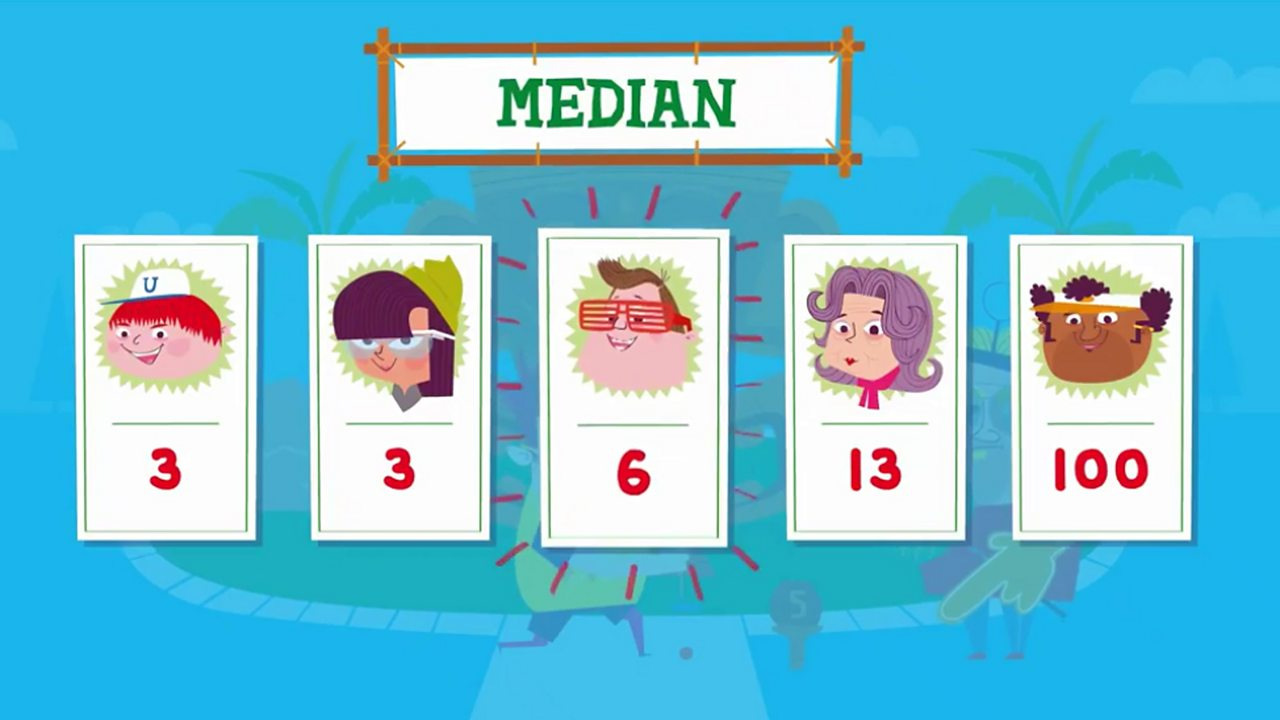News

# 8 of the Best Mean, Median and Mode Worksheets and Resources for KS3 and KS4 Maths

Sometimes it's good to be average, common and stuck in the middle, as your secondary students can find out with these lesson plans, activities, ideas and more...

•### 1 | Mean, median, mode and range definitionsAlways a good place to start to know the definitions of the terms you’re studying, so students can get a good reminder on the BBC Bitesize site.

It explains that:

• The median is the middle value
• The mode is the number that appears the most
• The mean is the total of the numbers divided by how many numbers there are (what we often call the average)
• The range is the difference between the biggest and the smallest number

It gives clear examples to help students understand, plus there are short videos and interactive questions.

Give it all a look here.

### 2 | Explanation and worksheetsFor a more in depth explanation, this one is designed for GCSE revision, and there is a selection of worksheets to download at the bottom of the page.

Find them all here.

### 3 | Understanding the meaning of the meanThe language of averages is used all the time in the world around us – and budding mathematicians need to understand exactly what such terms represent.

That’s the basis of this lesson plan from Colin Foster.

Check it out on our sister site teachsecondary.com.

### 4 | GCSE questionsThis worksheet features 20 GCSE questions (each with multiple parts) on mean, median, mode and range.

### 5 | Analysing and displaying dataThis Pearson resource is a colourful 29-page PDF covering data, that’s divided into six topics, the first of which is mean, median and mode.

There are also sections at the end on check up, extend, strengthen and test.

Get this resource here.

### 6 | TeachIt MathsHead to TeachIt Maths for a selection of mean, median and mode resources from posters to starter activities and word problems like the one pictured above.

Check out the full range here.

### 7 | Correcting Clumsy CliveThis resource from Andy Lutwuche features five more questions that his character, Clumsy Clive, seems a bit confused on. These involve median and mean from sets of data, plus calculating the mean from a frequency table and estimating the mean from grouped data.

You can also throw a couple more questions in verbally (find the modal group etc) while discussing the answers and reasons why as a class.

### 8 | Average and range codebreaker activitiesAnother one from Andy is this codebreaker that asks students to complete the calculations, solve the anagrams and find the punchline for a joke.

Get this one here.